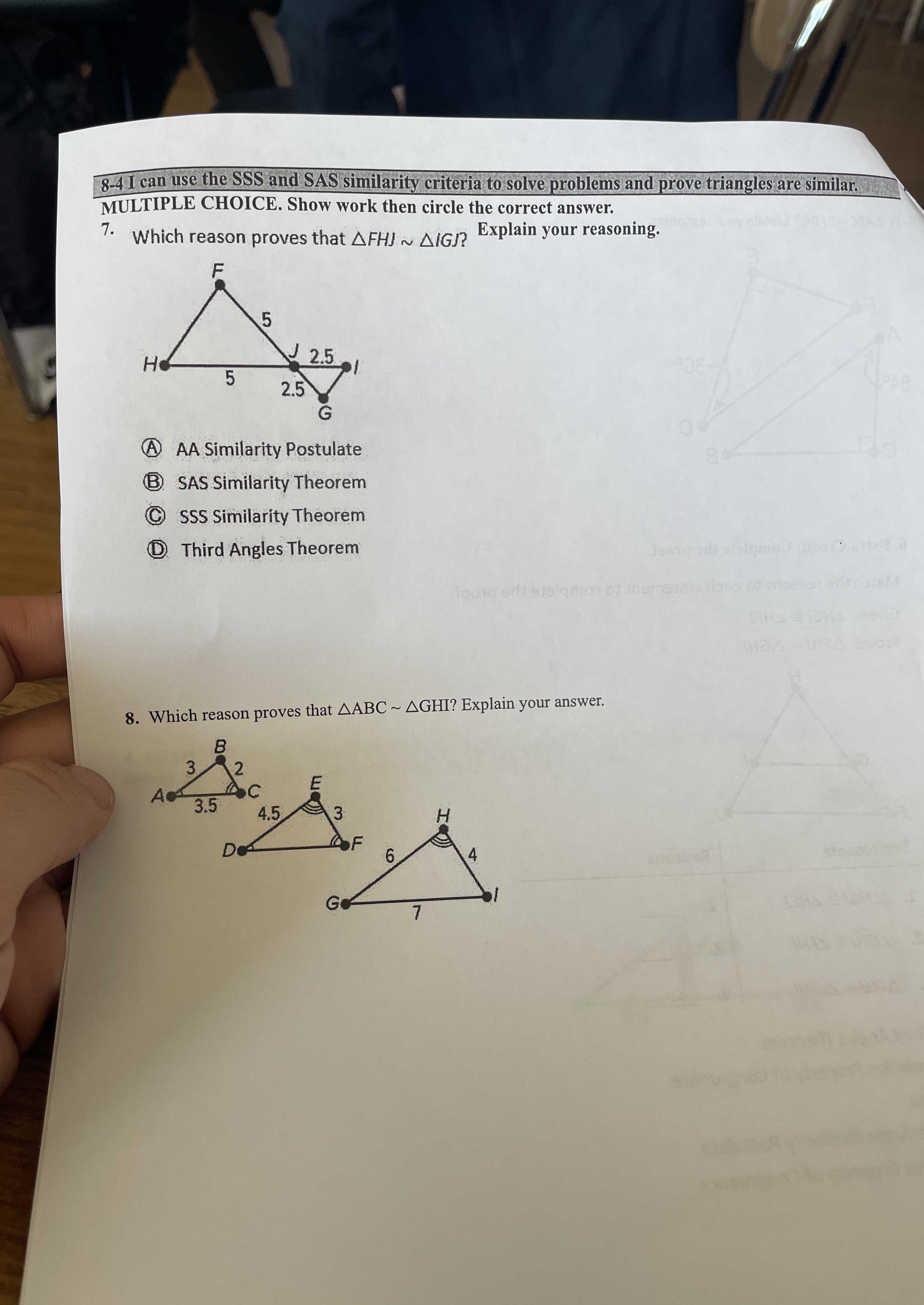### Still have math questions?

Trigonometry
Question7. Which reason proves that $$\triangle F H J \sim \Delta I G J$$ ?

(A) AA Similarity Postulate (B) SAS Similarity Theorem

(C) SSS Similarity Theorem (D) Third Angles Theorem

8. Which reason proves that $$\triangle ABC \sim \triangle GHI$$ ? Explain your answer.